## json字符串中key值驼峰命名与下划线命名互转

CloudArchitect 发布于 2016/10/26 14:18

1.把所有的key值用正则提取替换。感觉这种方式过于简单粗暴

2.把json字符串解析成json object 然后遍历key，生成目标的key与json串，但是这种方式涉及到key值嵌套递归问题，不好写

0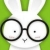```public static void main(String[] args) {
String str = "{'user_name':'ok','user_sex':0,object_info':{'business_code':'0001','object_info2':{'object_1':'ok'}}}";
String strs[] = str.split(":");
/*for (String is : strs) {
System.out.println(is);
}*/
StringBuilder sb = new StringBuilder();
for (String o : strs) {
String _tmp[]  = o.split(",");
if(_tmp.length > 1){
//替换数组2的值为驼峰
sb.append(_tmp).append(",").append(underlineToCamel(_tmp));
}else{
//替换''里的数值为驼峰
sb.append(underlineToCamel(_tmp));
}

sb.append(":");
}

System.out.println(sb.substring(0,sb.length() - 1).toString());
}

public static final char UNDERLINE='_';
public static String camelToUnderline(String param){
if (param==null||"".equals(param.trim())){
return "";
}
int len=param.length();
StringBuilder sb=new StringBuilder(len);
for (int i = 0; i < len; i++) {
char c=param.charAt(i);
if (Character.isUpperCase(c)){
sb.append(UNDERLINE);
sb.append(Character.toLowerCase(c));
}else{
sb.append(c);
}
}
return sb.toString();
}
public static String underlineToCamel(String param){
if (param==null||"".equals(param.trim())){
return "";
}
int len=param.length();
StringBuilder sb=new StringBuilder(len);
for (int i = 0; i < len; i++) {
char c=param.charAt(i);
if (c==UNDERLINE){
if (++i<len){
sb.append(Character.toUpperCase(param.charAt(i)));
}
}else{
sb.append(c);
}
}
return sb.toString();
}
public static String underlineToCamel2(String param){
if (param==null||"".equals(param.trim())){
return "";
}
StringBuilder sb=new StringBuilder(param);
Matcher mc= Pattern.compile("_").matcher(param);
int i=0;
while (mc.find()){
int position=mc.end()-(i++);
//String.valueOf(Character.toUpperCase(sb.charAt(position)));
sb.replace(position-1,position+1,sb.substring(position,position+1).toUpperCase());
}
return sb.toString();
}```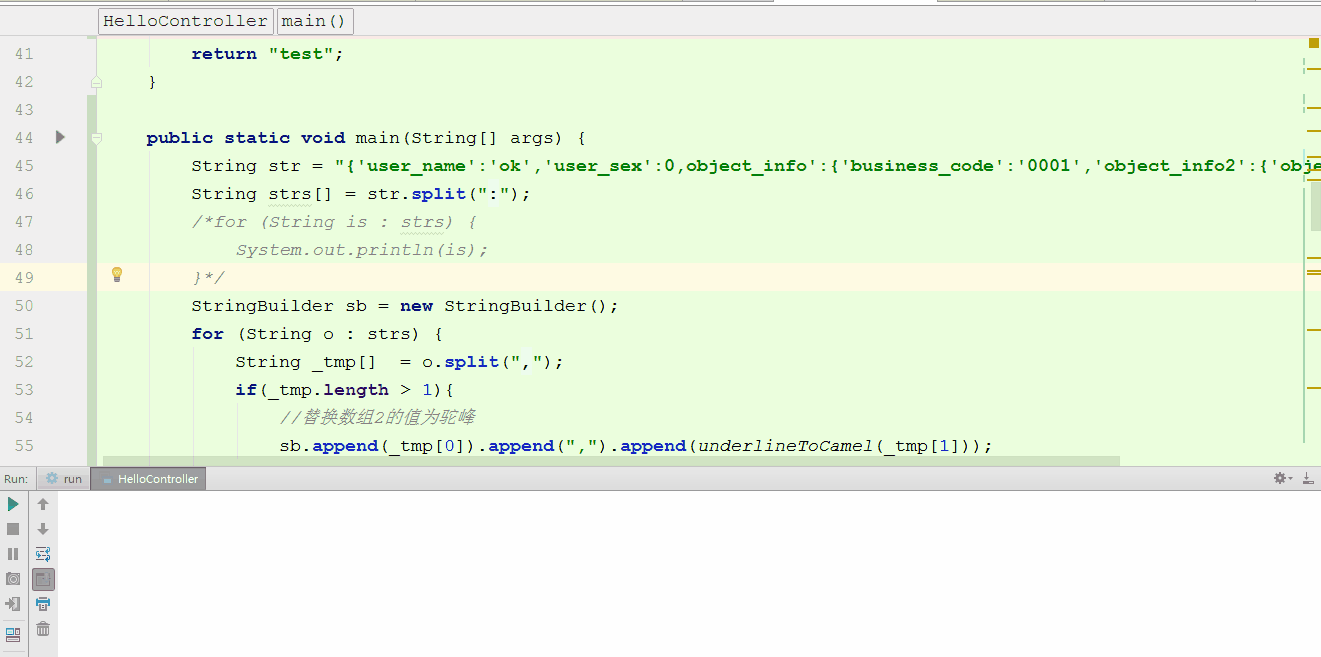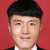0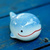0```    public final static Object convert(String json) {
Object obj = JSON.parse(json);
convert(obj);
return obj;
}

public final static void convert(Object json) {
if (json instanceof JSONArray) {
JSONArray arr = (JSONArray) json;
for (Object obj : arr) {
convert(obj);
}
} else if (json instanceof JSONObject) {
JSONObject jo = (JSONObject) json;
Set<String> keys = jo.keySet();
String[] array = keys.toArray(new String[keys.size()]);
for (String key : array) {
Object value = jo.get(key);
String[] key_strs = key.split("_");
if (key_strs.length > 1) {
StringBuilder sb = new StringBuilder();
for (int i = 0; i < key_strs.length; i++) {
String ks = key_strs[i];
if (!"".equals(ks)) {
if (i == 0) {
sb.append(ks);
} else {
int c = ks.charAt(0);
if (c >= 97 && c <= 122) {
int v = c - 32;
sb.append((char) v);
if (ks.length() > 1) {
sb.append(ks.substring(1));
}
} else {
sb.append(ks);
}
}
}
}
jo.remove(key);
jo.put(sb.toString(), value);
}
convert(value);
}
}
}```
0
d0#### 引用来自“BoXuan”的评论

```    public final static void convert(Object json) {
if (json instanceof JSONArray) {
JSONArray arr = (JSONArray) json;
for (Object obj : arr) {
convert(obj);
}
} else if (json instanceof JSONObject) {
JSONObject jo = (JSONObject) json;
Set<String> keys = jo.keySet();
//此处不能直接遍历keys，不然将报ConcurrentModificationException异常
String[] array = keys.toArray(new String[keys.size()]);
for (String key : array) {
Object value = jo.get(key);
String[] key_strs = key.split("_");
if (key_strs.length > 1) {
StringBuilder sb = new StringBuilder();
for (int i = 0; i < key_strs.length; i++) {
String ks = key_strs[i];
if (!"".equals(ks)) {
if (i == 0) {
sb.append(ks);
} else {
int c = ks.charAt(0);
if (c >= 97 && c <= 122) {
int v = c - 32;
sb.append((char) v);
if (ks.length() > 1) {
sb.append(ks.substring(1));
}
} else {
sb.append(ks);
}
}
}
jo.remove(key);
jo.put(sb.toString(), value);
}
convert(value);
}
}
}

public final static Object convert(String json) {
Object obj = JSON.parse(json);
convert(obj);
return obj;
}
```

```public final static void convert(Object json) {
if (json instanceof JSONArray) {
JSONArray arr = (JSONArray) json;
for (Object obj : arr) {
convert(obj);
}
} else if (json instanceof JSONObject) {
JSONObject jo = (JSONObject) json;
Set<String> keys = jo.keySet();
String[] array = new String[keys.size()];
int n = 0;
for(String key : keys) {
array[n] = key;
n++;
}
for (String key : array) {
Object value = jo.get(key);
jo.remove(key);
convert(value);
jo.put(underlineToCamel(key), value);
}
}
}```0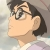0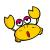0#### 引用来自“dwangel”的评论

```public class CameTools {

public static void main(String[] args) {
String str = "{'user_name':'o,k','user_sex':0,'object_info':{'business_code':'0001','object_info2':{'object_1':'ok'}}}";
String str2 = "{'object_info':{'business_code':'ok'}}";
System.out.println(convert(str));
}

public final static void convert(Object json) {
if (json instanceof JSONArray) {
JSONArray arr = (JSONArray) json;
for (Object obj : arr) {
convert(obj);
}
} else if (json instanceof JSONObject) {
JSONObject jo = (JSONObject) json;
Set<String> keys = jo.keySet();
//此处不能直接遍历keys，不然将报ConcurrentModificationException异常
String[] array = keys.toArray(new String[keys.size()]);
for (String key : array) {
Object value = jo.get(key);
String[] key_strs = key.split("_");
if (key_strs.length > 1) {
StringBuilder sb = new StringBuilder();
for (int i = 0; i < key_strs.length; i++) {
String ks = key_strs[i];
if (!"".equals(ks)) {
if (i == 0) {
sb.append(ks);
} else {
int c = ks.charAt(0);
if (c >= 97 && c <= 122) {
int v = c - 32;
sb.append((char) v);
if (ks.length() > 1) {
sb.append(ks.substring(1));
}
} else {
sb.append(ks);
}
}
}
jo.remove(key);
jo.put(sb.toString(), value);
}
convert(value);
}
}
}

}

public final static Object convert(String json) {
Object obj = JSONObject.fromObject(json);
convert(obj);
return obj;
}

}```

0#### 引用来自“szwx855”的评论

```public class CameTools {

public static void main(String[] args) {
String str = "{'user_name':'o,k','user_sex':0,'object_info':{'business_code':'0001','object_info2':{'object_1':'ok'}}}";
String str2 = "{'object_info':{'business_code':'ok'}}";
System.out.println(convert(str));
}

public final static void convert(Object json) {
if (json instanceof JSONArray) {
JSONArray arr = (JSONArray) json;
for (Object obj : arr) {
convert(obj);
}
} else if (json instanceof JSONObject) {
JSONObject jo = (JSONObject) json;
Set<String> keys = jo.keySet();
//此处不能直接遍历keys，不然将报ConcurrentModificationException异常
String[] array = keys.toArray(new String[keys.size()]);
for (String key : array) {
Object value = jo.get(key);
String[] key_strs = key.split("_");
if (key_strs.length > 1) {
StringBuilder sb = new StringBuilder();
for (int i = 0; i < key_strs.length; i++) {
String ks = key_strs[i];
if (!"".equals(ks)) {
if (i == 0) {
sb.append(ks);
} else {
int c = ks.charAt(0);
if (c >= 97 && c <= 122) {
int v = c - 32;
sb.append((char) v);
if (ks.length() > 1) {
sb.append(ks.substring(1));
}
} else {
sb.append(ks);
}
}
}
jo.remove(key);
jo.put(sb.toString(), value);
}
convert(value);
}
}
}

}

public final static Object convert(String json) {
Object obj = JSONObject.fromObject(json);
convert(obj);
return obj;
}

}```

```这个没问题

public class CameTools {
public static void main(String[] args) {
String str = "{'user_name':'o,k','user_sex':0,'object_info':{'business_code':'0001','object_info2':{'object_1':'ok'}}}";
String str2 = "{'object_info':{'business_code':'ok'}}";
System.out.println(convert(str2));
}

public final static void convert(Object json) {
if (json instanceof JSONArray) {
JSONArray arr = (JSONArray) json;
for (Object obj : arr) {
convert(obj);
}
} else if (json instanceof JSONObject) {
JSONObject jo = (JSONObject) json;
Set<String> keys = jo.keySet();
String[] array = keys.toArray(new String[keys.size()]);
for (String key : array) {
Object value = jo.get(key);
String[] key_strs = key.split("_");
if (key_strs.length > 1) {
StringBuilder sb = new StringBuilder();
for (int i = 0; i < key_strs.length; i++) {
String ks = key_strs[i];
if (!"".equals(ks)) {
if (i == 0) {
sb.append(ks);
} else {
int c = ks.charAt(0);
if (c >= 97 && c <= 122) {
int v = c - 32;
sb.append((char) v);
if (ks.length() > 1) {
sb.append(ks.substring(1));
}
} else {
sb.append(ks);
}
}
}
}
jo.remove(key);
jo.put(sb.toString(), value);
}
convert(value);
}
}
}

public final static Object convert(String json) {
Object obj = JSON.parse(json);
convert(obj);
return obj;
}

}```